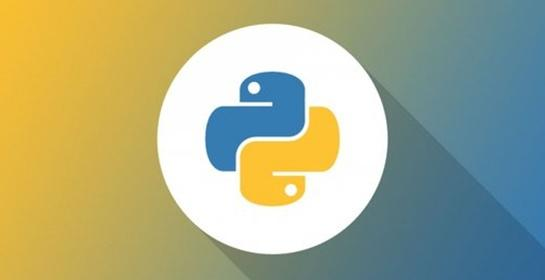• 写文章
• 提问

# python3时间中time是什么？怎么转换？• 时间戳，一般指Unix时间戳，是从1970年开始到现在的秒数。

• 本地时间的struct_time形式：一个长度为11的命名元组，第一位为年，第二位为月....

• UTC时间的struct_time形式：一个长度为11的命名元组，类似于上个，只不过为UTC时间

```print("time stamp:", time.time())         # 时间戳：1479193181.829338

print("local time:", time.localtime())    # struct_time类型的本地时间
time.struct_time(tm_year=2016, tm_mon=11, tm_mday=15, tm_hour=14, tm_min=59, tm_sec=41, tm_wday=1, tm_yday=320, tm_isdst=0)

print("utc time:", time.gmtime())         # struct_time类型的utc时间
time.struct_time(tm_year=2016, tm_mon=11, tm_mday=15, tm_hour=6, tm_min=59, tm_sec=41, tm_wday=1, tm_yday=320, tm_isdst=0)```

```time_stamp = time.time()               # 时间戳
local_time = time.localtime(time_stamp)# 时间戳转struct_time类型的本地时间
utc_time = time.gmtime(time_stamp)     # 时间戳转struct_time类型的utc时间

time_stamp_1 = time.mktime(local_time) # struct_time类型的本地时间转时间戳
time_stamp_2 = calendar.timegm(utc_time)# struct_time类型的utc时间转时间戳
print(time_stamp, time_stamp_1, time_stamp_2)```

```print(time.ctime(time_stamp))       # 时间戳转字符串(本地时间字符串)

print(time.asctime(local_time))     # struct_time类型的本地时间转字符串
print(time.asctime(utc_time))       # struct_time类型的utc时间转字符串

# struct_time类型的本地时间转字符串：自定义格式
print(time.strftime("%Y-%m-%d, %H:%M:%S, %w", local_time))
# struct_time类型的utc时间转字符串：自定义格式
print(time.strftime("%Y-%m-%d, %H:%M:%S, %w", utc_time))

struct_time = time.strptime("2016-11-15, 15:32:12, 2", "%Y-%m-%d, %H:%M:%S, %w")       # 字符串转struct_time类型```